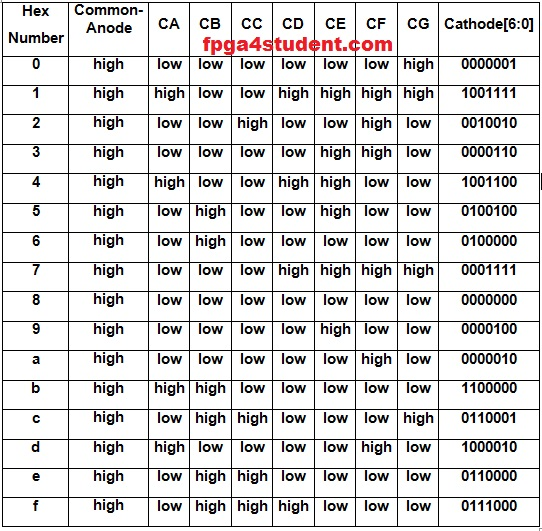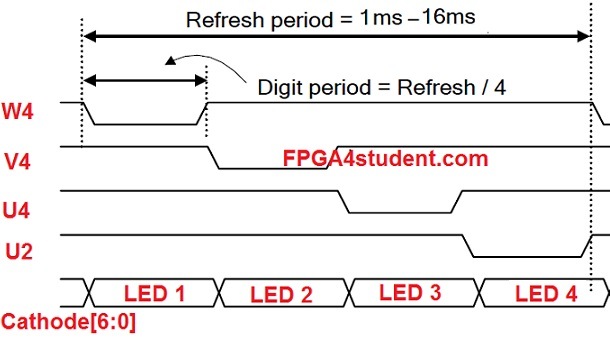# VHDL code for Seven-Segment Display on Basys 3 FPGA

## This VHDL project will present a full VHDL code for seven-segment display on Basys 3 FPGA. The seven-segment display on Basys 3 FPGA will be used to display a 4-digit hexadecimal number which is counting up every 1 second.First of all, the truth table for BCD to 7-segment display decoder on Basys 3 FPGA is as follows:### VHDL code for BCD to 7-segment display decoder:

`-- fpga4student: FPGA projects, Verilog projects, VHDL projects`
`-- Example VHDL code for BCD to seven-segment display on Basys 3 FPGA`
```process(LED_BCD)
begin
case LED_BCD is
when "0000" => LED_out <= "0000001"; -- "0"
when "0001" => LED_out <= "1001111"; -- "1"
when "0010" => LED_out <= "0010010"; -- "2"
when "0011" => LED_out <= "0000110"; -- "3"
when "0100" => LED_out <= "1001100"; -- "4"
when "0101" => LED_out <= "0100100"; -- "5"
when "0110" => LED_out <= "0100000"; -- "6"
when "0111" => LED_out <= "0001111"; -- "7"
when "1000" => LED_out <= "0000000"; -- "8"
when "1001" => LED_out <= "0000100"; -- "9"
when "1010" => LED_out <= "0000010"; -- a
when "1011" => LED_out <= "1100000"; -- b
when "1100" => LED_out <= "0110001"; -- C```
```    when "1101" => LED_out <= "1000010"; -- d
when "1110" => LED_out <= "0110000"; -- E
when "1111" => LED_out <= "0111000"; -- F
end case;
end process;
```

#### Next, as I mentioned in the tutorial, a seven-segment display controller must be used to control the 4-digit seven-segment display on Basys 3 FPGA.

Below is the timing diagram for refreshing the 4-digit seven-segment display on Basys 3 FPGA:The refresh rate needed for the 4-digit seven-segment display is from 1ms to 16ms. Let's choose 10.5ms as the refresh period. Below is an example VHDL code for creating refresh rate and anode signals for the 4-digit seven-segment display on Basys 3 FPGA:
```-- 7-segment display controller
-- generate refresh period of 10.5ms
process(clock_100Mhz,reset)
begin
if(reset='1') then
refresh_counter <= (others => '0');
elsif(rising_edge(clock_100Mhz)) then
refresh_counter <= refresh_counter + 1;
end if;
end process;
LED_activating_counter <= refresh_counter(19 downto 18);
-- 4-to-1 MUX to generate anode activating signals for 4 LEDs
process(LED_activating_counter)
begin
case LED_activating_counter is
when "00" =>
Anode_Activate <= "0111";
-- activate LED1 and Deactivate LED2, LED3, LED4
LED_BCD <= displayed_number(15 downto 12);
-- the first hex digit of the 16-bit number
when "01" =>
Anode_Activate <= "1011";
-- activate LED2 and Deactivate LED1, LED3, LED4
LED_BCD <= displayed_number(11 downto 8);
-- the second hex digit of the 16-bit number
when "10" =>
Anode_Activate <= "1101";
-- activate LED3 and Deactivate LED2, LED1, LED4
LED_BCD <= displayed_number(7 downto 4);
-- the third hex digit of the 16-bit number
when "11" =>
Anode_Activate <= "1110";
-- activate LED4 and Deactivate LED2, LED3, LED1
LED_BCD <= displayed_number(3 downto 0);
-- the fourth hex digit of the 16-bit number
end case;
end process;```

### VHDL code for seven-segment display on Bays 3 FPGA:

```-- fpga4student.com: FPGA projects, Verilog projects, VHDL projects
-- VHDL code for seven-segment display on Basys 3 FPGA
library IEEE;
use IEEE.STD_LOGIC_1164.ALL;
use IEEE.std_logic_unsigned.all;
entity seven_segment_display_VHDL is
Port ( clock_100Mhz : in STD_LOGIC;-- 100Mhz clock on Basys 3 FPGA board
reset : in STD_LOGIC; -- reset
Anode_Activate : out STD_LOGIC_VECTOR (3 downto 0);-- 4 Anode signals
LED_out : out STD_LOGIC_VECTOR (6 downto 0));-- Cathode patterns of 7-segment display
end seven_segment_display_VHDL;

architecture Behavioral of seven_segment_display_VHDL is
signal one_second_counter: STD_LOGIC_VECTOR (27 downto 0);
-- counter for generating 1-second clock enable
signal one_second_enable: std_logic;
-- one second enable for counting numbers
signal displayed_number: STD_LOGIC_VECTOR (15 downto 0);
-- counting decimal number to be displayed on 4-digit 7-segment display
signal LED_BCD: STD_LOGIC_VECTOR (3 downto 0);
signal refresh_counter: STD_LOGIC_VECTOR (19 downto 0);
-- creating 10.5ms refresh period
signal LED_activating_counter: std_logic_vector(1 downto 0);
-- the other 2-bit for creating 4 LED-activating signals
-- count         0    ->  1  ->  2  ->  3
-- activates    LED1    LED2   LED3   LED4
-- and repeat
begin
-- VHDL code for BCD to 7-segment decoder
-- Cathode patterns of the 7-segment LED display
process(LED_BCD)
begin
case LED_BCD is
when "0000" => LED_out <= "0000001"; -- "0"
when "0001" => LED_out <= "1001111"; -- "1"
when "0010" => LED_out <= "0010010"; -- "2"
when "0011" => LED_out <= "0000110"; -- "3"
when "0100" => LED_out <= "1001100"; -- "4"
when "0101" => LED_out <= "0100100"; -- "5"
when "0110" => LED_out <= "0100000"; -- "6"
when "0111" => LED_out <= "0001111"; -- "7"
when "1000" => LED_out <= "0000000"; -- "8"
when "1001" => LED_out <= "0000100"; -- "9"
when "1010" => LED_out <= "0000010"; -- a```
```    when "1011" => LED_out <= "1100000"; -- b
when "1100" => LED_out <= "0110001"; -- C
when "1101" => LED_out <= "1000010"; -- d
when "1110" => LED_out <= "0110000"; -- E
when "1111" => LED_out <= "0111000"; -- F
end case;
end process;
-- 7-segment display controller
-- generate refresh period of 10.5ms
process(clock_100Mhz,reset)
begin
if(reset='1') then
refresh_counter <= (others => '0');
elsif(rising_edge(clock_100Mhz)) then
refresh_counter <= refresh_counter + 1;
end if;
end process;
LED_activating_counter <= refresh_counter(19 downto 18);
-- 4-to-1 MUX to generate anode activating signals for 4 LEDs
process(LED_activating_counter)
begin
case LED_activating_counter is
when "00" =>
Anode_Activate <= "0111";
-- activate LED1 and Deactivate LED2, LED3, LED4
LED_BCD <= displayed_number(15 downto 12);
-- the first hex digit of the 16-bit number
when "01" =>
Anode_Activate <= "1011";
-- activate LED2 and Deactivate LED1, LED3, LED4
LED_BCD <= displayed_number(11 downto 8);
-- the second hex digit of the 16-bit number
when "10" =>
Anode_Activate <= "1101";
-- activate LED3 and Deactivate LED2, LED1, LED4
LED_BCD <= displayed_number(7 downto 4);
-- the third hex digit of the 16-bit number
when "11" =>
Anode_Activate <= "1110";
-- activate LED4 and Deactivate LED2, LED3, LED1
LED_BCD <= displayed_number(3 downto 0);
-- the fourth hex digit of the 16-bit number
end case;
end process;
-- Counting the number to be displayed on 4-digit 7-segment Display
-- on Basys 3 FPGA board
process(clock_100Mhz, reset)
begin
if(reset='1') then
one_second_counter <= (others => '0');
elsif(rising_edge(clock_100Mhz)) then
if(one_second_counter>=x"5F5E0FF") then
one_second_counter <= (others => '0');
else
one_second_counter <= one_second_counter + "0000001";
end if;
end if;
end process;
one_second_enable <= '1' when one_second_counter=x"5F5E0FF" else '0';
process(clock_100Mhz, reset)
begin
if(reset='1') then
displayed_number <= (others => '0');
elsif(rising_edge(clock_100Mhz)) then
if(one_second_enable='1') then
displayed_number <= displayed_number + x"0001";
end if;
end if;
end process;
end Behavioral;
```

#### Pin assignment constraint file for the 4-digit seven-segment display on Basys 3 FPGA:

```# Clock signal
set_property PACKAGE_PIN W5 [get_ports clock_100Mhz]
set_property IOSTANDARD LVCMOS33 [get_ports clock_100Mhz]
set_property PACKAGE_PIN R2 [get_ports reset]
set_property IOSTANDARD LVCMOS33 [get_ports reset]
#seven-segment LED display
set_property PACKAGE_PIN W7 [get_ports {LED_out}]
set_property IOSTANDARD LVCMOS33 [get_ports {LED_out}]
set_property PACKAGE_PIN W6 [get_ports {LED_out}]
set_property IOSTANDARD LVCMOS33 [get_ports {LED_out}]
set_property PACKAGE_PIN U8 [get_ports {LED_out}]
set_property IOSTANDARD LVCMOS33 [get_ports {LED_out}]
set_property PACKAGE_PIN V8 [get_ports {LED_out}]
set_property IOSTANDARD LVCMOS33 [get_ports {LED_out}]
set_property PACKAGE_PIN U5 [get_ports {LED_out}]
set_property IOSTANDARD LVCMOS33 [get_ports {LED_out}]
set_property PACKAGE_PIN V5 [get_ports {LED_out}]
set_property IOSTANDARD LVCMOS33 [get_ports {LED_out}]
set_property PACKAGE_PIN U7 [get_ports {LED_out}]
set_property IOSTANDARD LVCMOS33 [get_ports {LED_out}]
set_property PACKAGE_PIN U2 [get_ports {Anode_Activate}]
set_property IOSTANDARD LVCMOS33 [get_ports {Anode_Activate}]
set_property PACKAGE_PIN U4 [get_ports {Anode_Activate}]
set_property IOSTANDARD LVCMOS33 [get_ports {Anode_Activate}]
set_property PACKAGE_PIN V4 [get_ports {Anode_Activate}]
set_property IOSTANDARD LVCMOS33 [get_ports {Anode_Activate}]
set_property PACKAGE_PIN W4 [get_ports {Anode_Activate}]
set_property IOSTANDARD LVCMOS33 [get_ports {Anode_Activate}]
```

#### 11 comments:

1.How to make a testbench for this project? any help would be appreciated.

1.Simply generate clock and reset in the testbench, then observe the simulation waveform. That's it.

2.Nope, doesnt work. Only thing you get to see is the clock and the reset. When you add other objects the values of them are "U" or "XXXXX"
Please upload your testbench.

2.how to do counter down

1.simply change from displayed_number <= displayed_number + x"0001" to displayed_number <= displayed_number - x"0001";

3.4.How to count until 10?

5.Hello,I would like to know how to change the frequency and refresh rate?
for like 1-second clock to 100Hz , 10.5ms to slightly faster.
Thanks.

6.How to write the same code using structure model?

7.Please tell me how to write the same code using structure modelling

8.does this work for DE0-Nano?